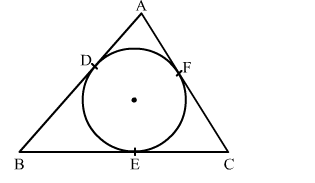# In the given figure, a circle inscribed in a triangle ABC, touches the sides AB, BC and AC at point D, EQuestion:

In the given figure, a circle inscribed in a triangle ABC, touches the sides AB, BC and AC at point D, E and F respectively. If AB = 12 cm, BC = 8 cm and AC = 10 cm, find the lengths of AD, BE and CFSolution:

We know that tangent segments to a circle from the same external point are congruent.
Now, we have
AD = AF, BD = BE and CE = CF
Now, AD + BD = 12 cm          .....(1)
AF + FC = 10 cm
⇒ AD + FC = 10 cm                    .....(2)
BE + EC = 8 cm
⇒ BD + FC = 8 cm                   .....(3)
AD + BD + AD + FC + BD + FC = 30
⇒2(AD + BD + FC) = 30
⇒AD + BD + FC = 15 cm           .....(4)
Solving (1) and (4), we get
FC = 3 cm
Solving (2) and (4), we get
BD = 5 cm
Solving (3) and (4), we get